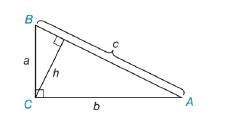Chapter 8.1, Problem 34EElementary Geometry For College St...

7th Edition
Alexander + 2 others
ISBN: 9781337614085

Solutions

Chapter
SectionElementary Geometry For College St...

7th Edition
Alexander + 2 others
ISBN: 9781337614085
Textbook Problem

In Exercises 34 to 36, provide paragraph proofs. Given: Right △ A B C Prove: h = a b cTo determine

To prove:

h=abc in a right ΔABC.

Explanation

1) Formula:

Area of the right triangle A=ab unit2.

Where, a and b are the two legs of the right triangle.

Area of the triangle A=bh unit2.

Where, b is the base of the triangle, and h is the height of the triangle.

2) Calculation:

Given,

By the figure,

a and b are the two legs and hypotenuse c of the triangle ΔABC

Area of the right triangle ABC=12ab unit2

(i.e.) AABC=12ab unit2(1)

When, AB¯ is the base of the triangle ΔABC

h is the height of the triangle and AB¯=c is base (b) of the triangle

Still sussing out bartleby?

Check out a sample textbook solution.

See a sample solution

The Solution to Your Study Problems

Bartleby provides explanations to thousands of textbook problems written by our experts, many with advanced degrees!

Get Started

What is the lowest score in the following distribution?

Essentials of Statistics for The Behavioral Sciences (MindTap Course List)

In Exercises 1-6, simplify the expression. 5. (4x1)(3)(3x+1)(4)(4x1)2

Applied Calculus for the Managerial, Life, and Social Sciences: A Brief Approach

In Problems 1 and 2, factor out the common monomial factor. 2.

Mathematical Applications for the Management, Life, and Social Sciences

Expand each expression in Exercises 122. (2x2)(3x4)

Finite Mathematics and Applied Calculus (MindTap Course List)

True or False: The function y = f(x) graphed at the right could be a solution to y = x + y.

Study Guide for Stewart's Single Variable Calculus: Early Transcendentals, 8th

True or False: converges absolutely.

Study Guide for Stewart's Multivariable Calculus, 8th